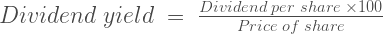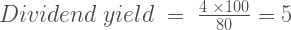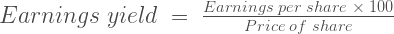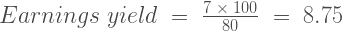# Dividend yield and earnings yield

March 28th, 2013

Calculating the dividend yield or the earnings yield of a stock and comparing it to the yield on government securities is one way of checking whether the price of a stock represents value. There is a difference between the dividend yield and the earnings yield of a stock.

## Dividend yield

The formula for calculating the dividend yield of a stock in percentage terms is:$Dividend\;yield\;=\;\frac{Dividend\;per\;share\;\times100}{Price\;of\;share}$

### Example 1

Suppose that the current price of a share is $80 and its annual dividend rate is$4. The dividend yield then is calculated like this:$Dividend\;yield\;=\;\frac{4\;\times100}{80}=5$

So we would say here that the shares in this company are yielding 5.00% at the current price.

## Earnings yield

As a general rule, companies do not return all their earnings in a given year to shareholders. Instead they pay out a lesser amount. As a result, the earnings yield of a share and the dividend yield of a share are usually different. The formula for calculating the earnings yield in percentage terms of a stock is:$Earnings\;yield\;=\;\frac{Earnings\;per\;share\;\times\;100}{Price\;of\;share}$

### Example 2

Suppose that the current price of a share is $80 and its annual earnings rate is$7. The earnings yield  then is calculated like this:$Earnings\;yield\;=\;\frac{7\; \times\;100}{80}\;=\;8.75$

So we would say here that the shares in this company are yielding an earnings rate of 8.75% at the current price.

To a great extent this is a reflection of the price/earnings ratio (P/E ratio) of a stock. If the above stock is selling at $80 and is earning$7 a share then, if you apply the P/E ratio formula, the answer is 11.42 (80/7). This means that a P/E ratio of 11.42 implies an earnings yield of 8.75 and vice versa.

## Using dividend yield to calculate the value of a share

Benjamin Graham placed a lot of emphasis on the dividend rates that a company pays and the consistency of payments. Warren Buffett, because he believes so strongly that owning a share in the company is the same as owning a tiny part of the business that the company is in, focuses on the earnings per share that a company gives him for his investment.

Buffett has a concept that he calls owner earnings which, even though it is more complex than earnings yield, has as its basis the earnings of the company rather than the dividends that it pays. Graham argues that a consistent record of paying dividends is one tool in the process of investment for the defensive investor.

Buffett’s concern about dividends relates to capital allocation. If company management can put surplus earnings to good use by growing earnings at a rate faster than one would expect the average investor to grow the dividends received, then that is the better use for the money. If company management cannot do this, it should give the money to shareholders by buying back shares (where the price of the shares does not exceed intrinsic value), by a return of capital or by payment of dividends (generally the least effective option tax-wise). Because of this, he values companies on what they earn and not on what they pay out as dividends.

Both Graham and Buffett believe that a basis for stock investment is whether buying the shares in a company are likely, taking added risk into account, to be a more profitable investment than a prime commercial bond (Graham) or government securities (Buffett).

For example, if the shares in a company with low growth prospects are yielding little more (dividend yield or earnings yield, as you prefer) than that available in a high quality bond (government or triple A rated), why would you take the extra risk of a share investment? If however the yield from a company is greater than bond yields, or you are confident that  its earnings and dividends will rise over a period, and there is little risk of the company business model failing, you would get a better return for your money bu buying the shares.

### Example 3 Comparing dividend yield to government bond yield

In Example 1, we worked out that the dividend yield on shares in that particular company is 5 per cent. At the time of writing this post, 10 year US Treasury Bills can be bought, yielding a rate of about 2.8 per cent. The dividend yield per share in Example 1, at 5 per cent, is almost double the rate available from the government bond. So, all things being equal, the stock investment seems preferable.

## Using earnings yield to calculate the value of a share

We can apply the same approach to earnings per share.

### Example 4 Comparing earnings yield to government bond yield

In Example 2, we worked out that the earnings yield on shares in that particular company is 8.75 per cent. At the time of writing this post, 10 year US Treasury Bills can be bought yielding a rate of about 2.8 per cent. The earnings yield per share in Example 2 at 8.75 per cent is more than three times the rate on the government bonds. So, all things being equal, the stock investment seems preferable.

## All things are not equal

In making a comparison between the yield available on a common stock, whether earnings or dividend, and the yield available on a government bond or a triple A commercial bond using these methods, the prudent investor would need to make a risk assessment. This is because we are comparing an investment that carries risk and uncertainty (shares) with an investment that is predictable in its yield and virtually risk free (government bond) or low risk (triple A commercial bond). An investor would need to consider:

• whether bond rates are likely to rise in the near future, in which case if the investor needs to sell before maturity, a capital loss is possible (the reverse would apply if bond rates were to fall).
• the potential for the earnings of that company to grow (in which case its attractiveness will increase in comparison to the fixed return of the bond) or diminish (the reverse situation).
• the risk of failure of the company or its business model, or overall economic deterioration.

## Using dividend yield to to calculate discounted cash flow (DCF)

If you are adept at higher mathematics, you can look at a paper written by Dr Aswath Damodoran, Professor of Finance at the Stern School at NYU.